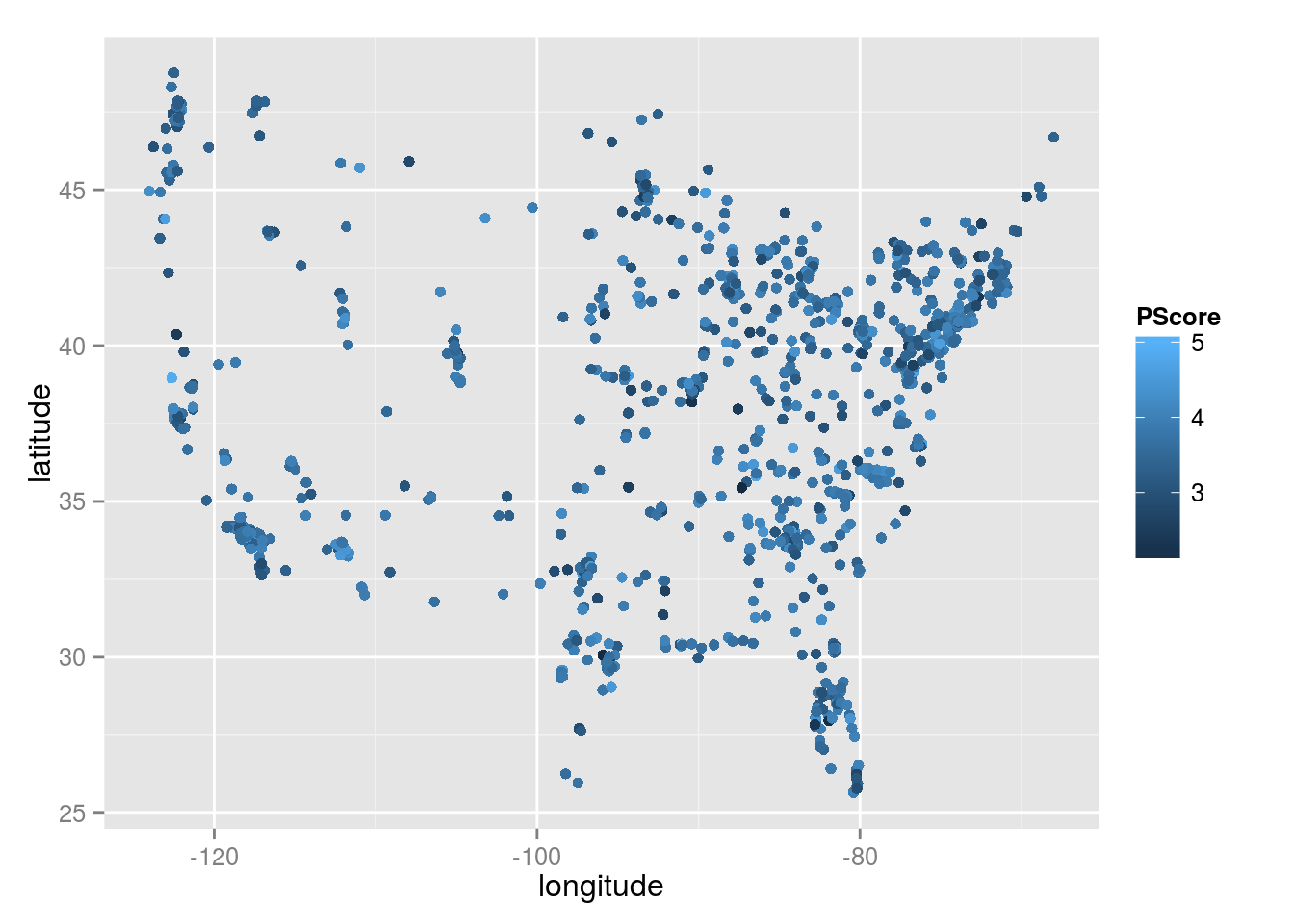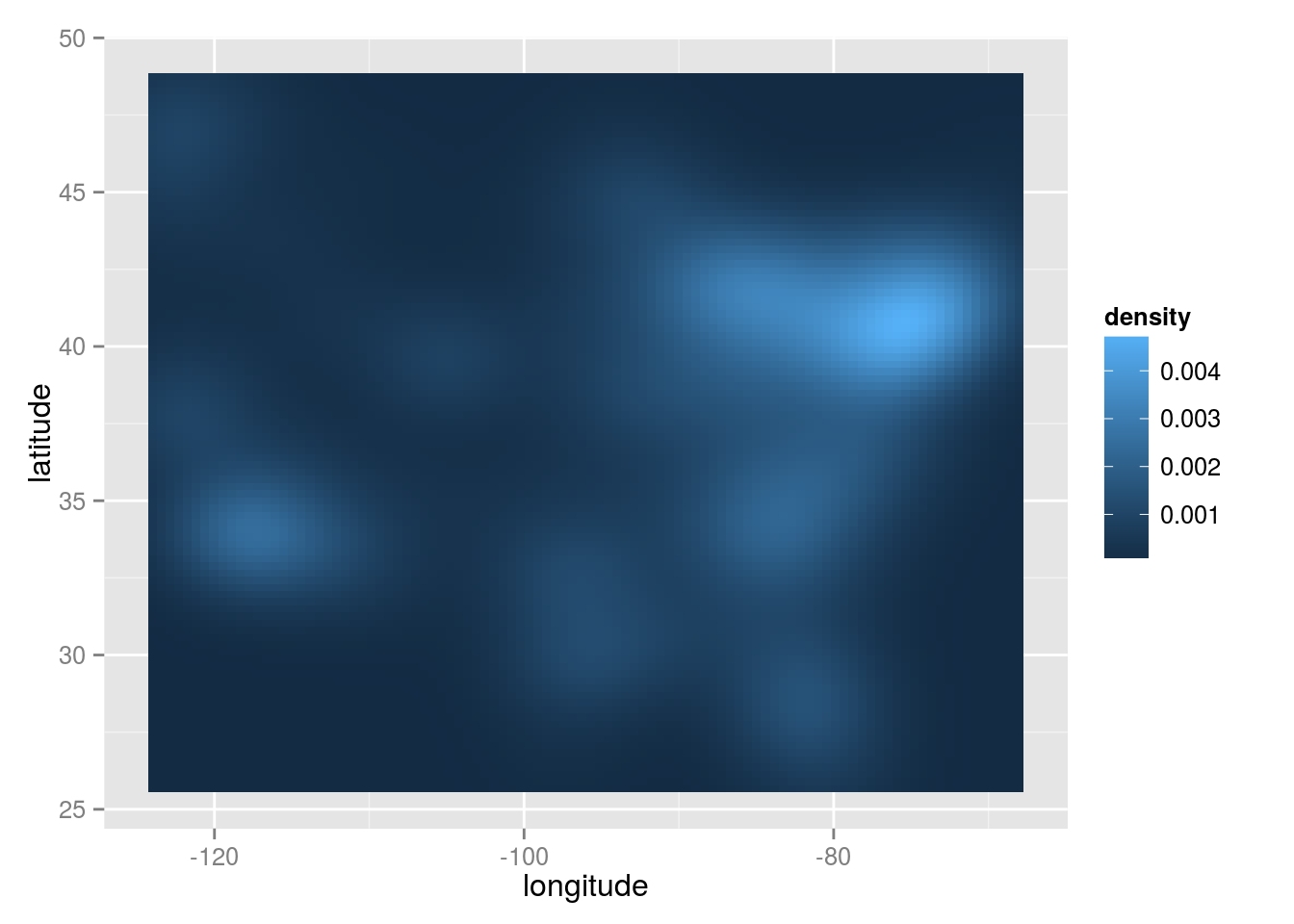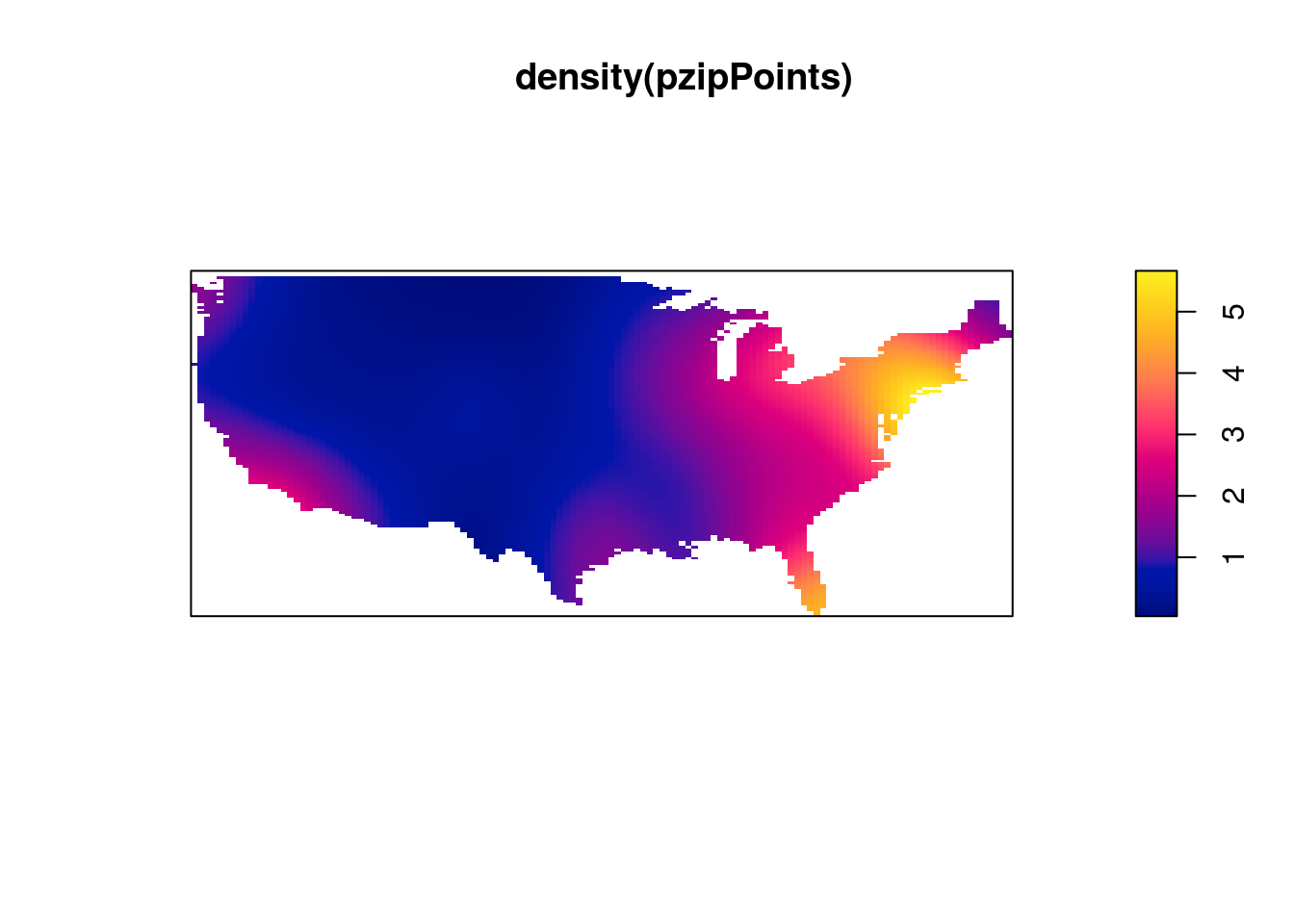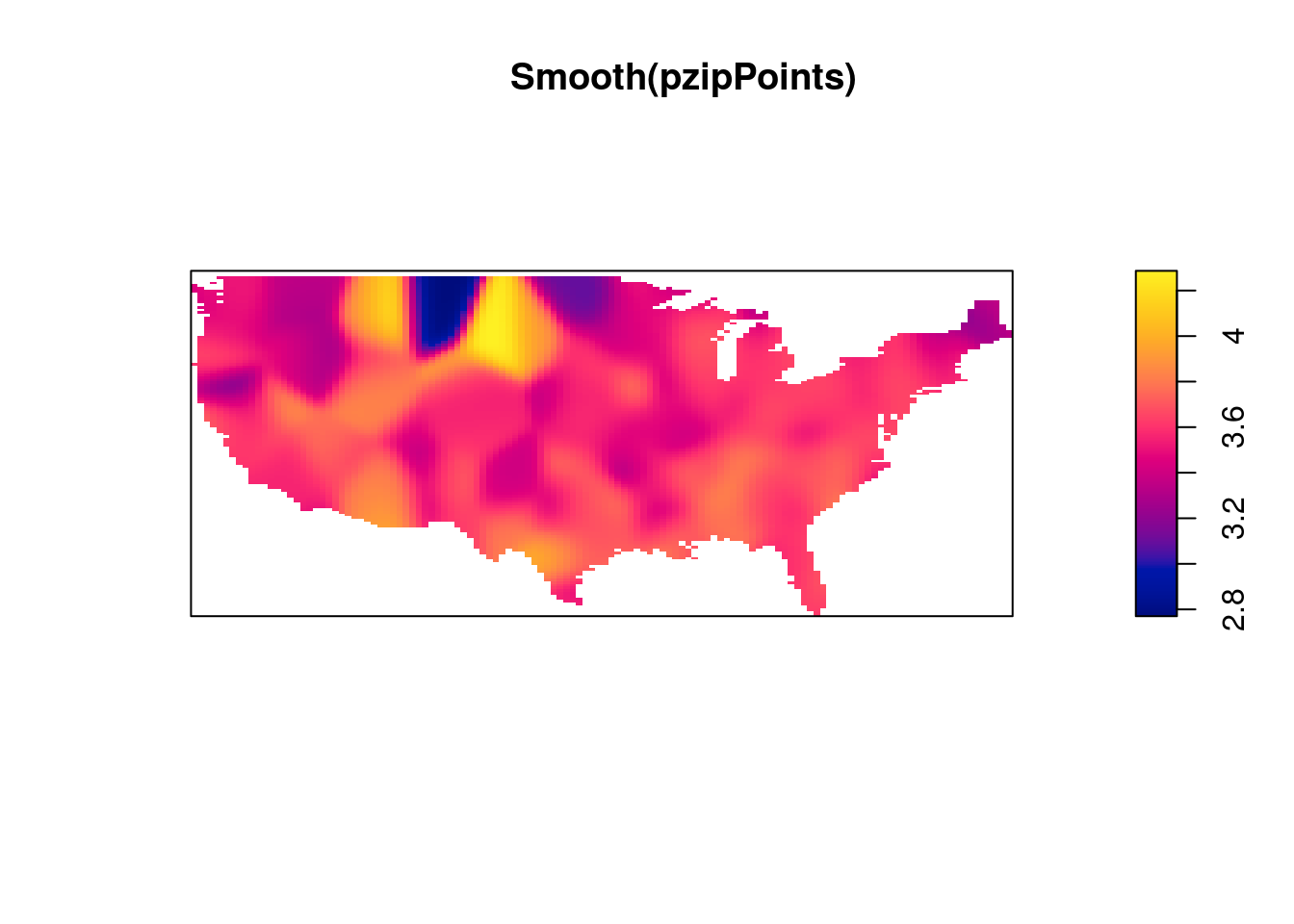# Dealing with “missing”/out of bounds values in heatmaps

I was tinkering around in R to see if I could plot better looking heatmaps, when I encountered an issue regarding how specific values are represented in plots with user-specified restricted ranges. When I’m plotting heatmaps, I usually want to restrict the range of the data being plotted in a specific way. In this case, I was plotting classifier accuracy across frequencies and time of Fourier-transformed EEG data, and I want to restrict the lowest value of the heatmap to be chance level (in this case, 50%).

I used the following code to generate a simple heatmap:

decoding <- readMat("/Users/Pablo/Desktop/testDecoding.mat")$plotData plotDecoding <- melt(decoding) quartz(width=5,height=4)  ggplot(plotDecoding, aes(x = Var2, y = Var1)) +  geom_tile(aes(fill = value)) +  scale_fill_viridis(name = "",limits = c(0.5,0.75)) And this is what I got:See the gray areas? What’s happening here is that any data points that fall outside of my specified data range (see the limits argument in scale_fill_viridis) are being converted to NAs. Apparently, NA values are plotted as gray by default. Obviously these values are not missing in the actual data; how can we actually ensure that they're plotted? The solution comes from the scales package that comes with ggplot. Whenever you create a plot with specified limits, include the argument oob = squish (oob = out of bounds) in the same line where you set the limits (make sure that the scales package is loaded). Ex: scale_fill_viridis(name = "",limits = c(0.5,0.75),oob=squish)  What this does is that values that fall below the specified range are represented as the minimum value (color, in this case), and values that fall above the range are represented as the maximum value (which seems to be what Matlab defaults to). The resulting heatmap looks like this:A simple solution to an annoying little quirk in R! # Smoothing the map ## Other ways to plot spatial data… Here, I walk through some ggplot methods, and finally introduce the spatstat package, which allows one to plot smoothed heatmaps based on your variable of interest. ### Basica Data Wrangling library(zipcode) library(dplyr) library(ggplot2) data(zipcode) pzip<-read.csv('StudyFiveSampleOneWaveOne_forRclub.csv') str(pzip) ## 'data.frame': 1192 obs. of 2 variables: ##$ ZipCode: int  23231 85712 85281 23917 NA 84337 89104 60629 80503 43449 ...
##  $PScore : num 3.37 4.3 2.97 3.2 2.9 ... pzip_clean<-pzip %>% mutate(zip=clean.zipcodes(ZipCode)) str(pzip_clean) ## 'data.frame': 1192 obs. of 3 variables: ##$ ZipCode: int  23231 85712 85281 23917 NA 84337 89104 60629 80503 43449 ...
##  $PScore : num 3.37 4.3 2.97 3.2 2.9 ... ##$ zip    : chr  "23231" "85712" "85281" "23917" ...
pzip_latlong <- left_join(pzip_clean,zipcode) %>%
filter(longitude > -140) %>%
na.omit
## Joining by: "zip"
str(pzip_latlong)
## 'data.frame':    1080 obs. of  7 variables:
##  $ZipCode : int 23231 85712 85281 23917 84337 89104 60629 80503 43449 93030 ... ##$ PScore   : num  3.37 4.3 2.97 3.2 3.17 ...
##  $zip : chr "23231" "85712" "85281" "23917" ... ##$ city     : chr  "Richmond" "Tucson" "Tempe" "Boydton" ...
##  $state : chr "VA" "AZ" "AZ" "VA" ... ##$ latitude : num  37.5 32.2 33.4 36.6 41.7 ...
##  $longitude: num -77.4 -110.9 -111.9 -78.3 -112.2 ... ### Points and Density Close your eyes and infer the shape of the nation…. pzip_latlong %>% filter(longitude > -140) %>% ggplot(aes(y=latitude,x=longitude)) + geom_point(aes(color=PScore))pzip_latlong %>% filter(longitude > -140) %>% ggplot(aes(y=latitude,x=longitude)) + stat_density2d(geom="tile", aes(fill = ..density..), contour = FALSE)### Spatially smoothed data on an actual map library(spatstat) library(maps) library(maptools) #map('usa') #points(pzip_latlong$longitude, pzip_latlong$latitude) usmap <- map('usa', fill=TRUE, col="transparent", plot=FALSE) uspoly <- map2SpatialPolygons(usmap, IDs=usmap$names,
proj4string=CRS("+proj=longlat +datum=wgs84"))
spatstat.options(checkpolygons=FALSE)
## Warning: spatstat.options('checkpolygons') will be ignored in future
## versions of spatstat
usowin <- as.owin.SpatialPolygons(uspoly)
spatstat.options(checkpolygons=TRUE)
## Warning: spatstat.options('checkpolygons') will be ignored in future
## versions of spatstat
pzip_noDupes <- pzip_latlong %>%
group_by(latitude, longitude) %>%
summarise(pscore=mean(PScore))

pzipPoints <- ppp(x=pzip_noDupes$longitude, y=pzip_noDupes$latitude,
marks=pzip_noDupes$pscore, window=usowin) ## Warning: point-in-polygon test had difficulty with 1 point (total score not ## 0 or 1) ## Warning in ppp(x = pzip_noDupes$longitude, y = pzip_noDupes$latitude, ## marks = pzip_noDupes$pscore, : 18 points were rejected as lying outside the
## specified window
plot(density(pzipPoints))plot(Smooth(pzipPoints))